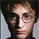# Fisher Transform Indicator by Ehlers - Strategy

26645 lượt xem
26645
Market prices do not have a Gaussian probability density function
as many traders think. Their probability curve is not bell-shaped.
But trader can create a nearly Gaussian PDF for prices by normalizing
them or creating a normalized indicator such as the relative strength
index and applying the Fisher transform . Such a transformed output
creates the peak swings as relatively rare events.
Fisher transform formula is: y = 0.5 * ln ((1+x)/(1-x))
The sharp turning points of these peak swings clearly and unambiguously
identify price reversals in a timely manner.
```////////////////////////////////////////////////////////////
//  Copyright by HPotter v1.0 01/07/2014
// 	Market prices do not have a Gaussian probability density function
// 	as many traders think. Their probability curve is not bell-shaped.
// 	But trader can create a nearly Gaussian PDF for prices by normalizing
// 	them or creating a normalized indicator such as the relative strength
// 	index and applying the Fisher transform. Such a transformed output
// 	creates the peak swings as relatively rare events.
// 	Fisher transform formula is: y = 0.5 * ln ((1+x)/(1-x))
// 	The sharp turning points of these peak swings clearly and unambiguously
// 	identify price reversals in a timely manner.
////////////////////////////////////////////////////////////
study(title="Fisher Transform Indicator by Ehlers Strategy", shorttitle="Fisher Transform Indicator by Ehlers")
Length = input(10, minval=1)
xHL2 = hl2
xMaxH = highest(xHL2, Length)
xMinL = lowest(xHL2,Length)
nValue1 = 0.33 * 2 * ((xHL2 - xMinL) / (xMaxH - xMinL) - 0.5) + 0.67 * nz(nValue1)
nValue2 = iff(nValue1 > .99,  .999,
iff(nValue1 < -.99, -.999, nValue1))
nFish = 0.5 * log((1 + nValue2) / (1 - nValue2)) + 0.5 * nz(nFish)
pos =	iff(nFish > nz(nFish), 1,
iff(nFish < nz(nFish), -1, nz(pos, 0)))
barcolor(pos == -1 ? red: pos == 1 ? green : blue )
plot(nFish, color=green, title="Fisher")
plot(nz(nFish), color=red, title="Trigger")```
DONATE/TIP

BTC: 3FKWwtaYrf5NHZzaCi1fyAfQj7XSgtyCqe